Скачать презентацию Business Statistics A First Course 5 th Edition

3b6f1153455be35353ae8e288a9f9e74.ppt

• Количество слайдов: 62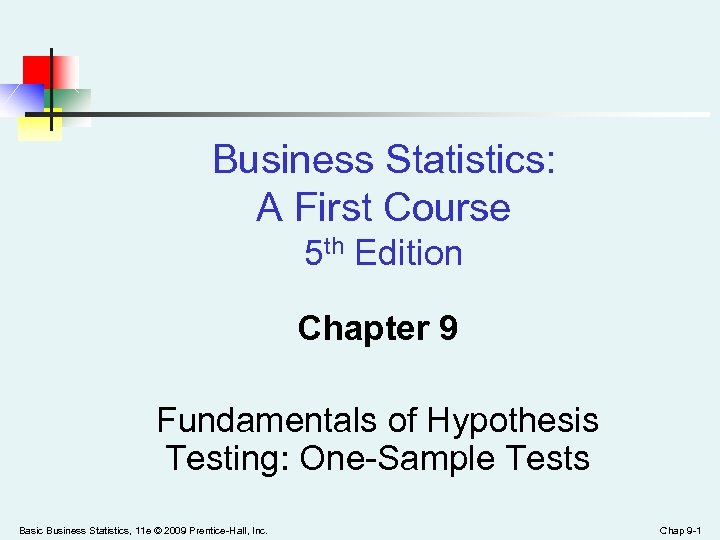Business Statistics: A First Course 5 th Edition Chapter 9 Fundamentals of Hypothesis Testing: One-Sample Tests Basic Business Statistics, 11 e © 2009 Prentice-Hall, Inc. Chap 9 -1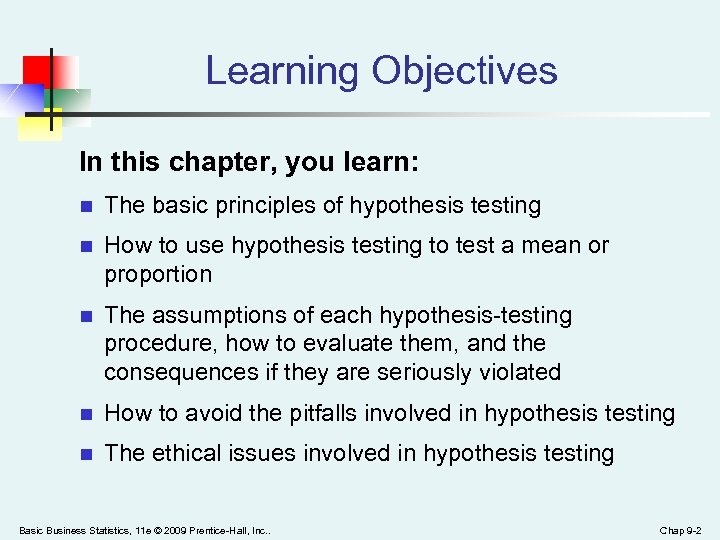Learning Objectives In this chapter, you learn: n The basic principles of hypothesis testing n How to use hypothesis testing to test a mean or proportion n The assumptions of each hypothesis-testing procedure, how to evaluate them, and the consequences if they are seriously violated n How to avoid the pitfalls involved in hypothesis testing n The ethical issues involved in hypothesis testing Basic Business Statistics, 11 e © 2009 Prentice-Hall, Inc. . Chap 9 -2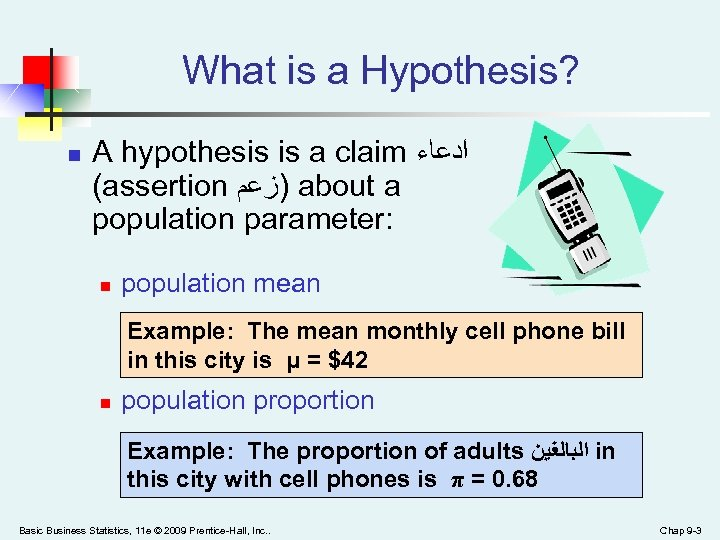What is a Hypothesis? n A hypothesis is a claim ﺍﺩﻋﺎﺀ (assertion )ﺯﻋﻢ about a population parameter: n population mean Example: The mean monthly cell phone bill in this city is μ = \$42 n population proportion Example: The proportion of adults ﺍﻟﺒﺎﻟﻐﻴﻦ in this city with cell phones is π = 0. 68 Basic Business Statistics, 11 e © 2009 Prentice-Hall, Inc. . Chap 9 -3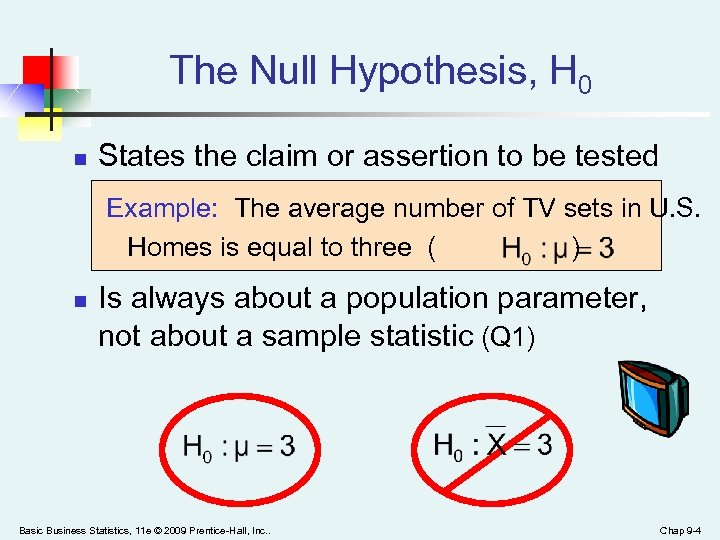The Null Hypothesis, H 0 n States the claim or assertion to be tested Example: The average number of TV sets in U. S. Homes is equal to three ( ) n Is always about a population parameter, not about a sample statistic (Q 1) Basic Business Statistics, 11 e © 2009 Prentice-Hall, Inc. . Chap 9 -4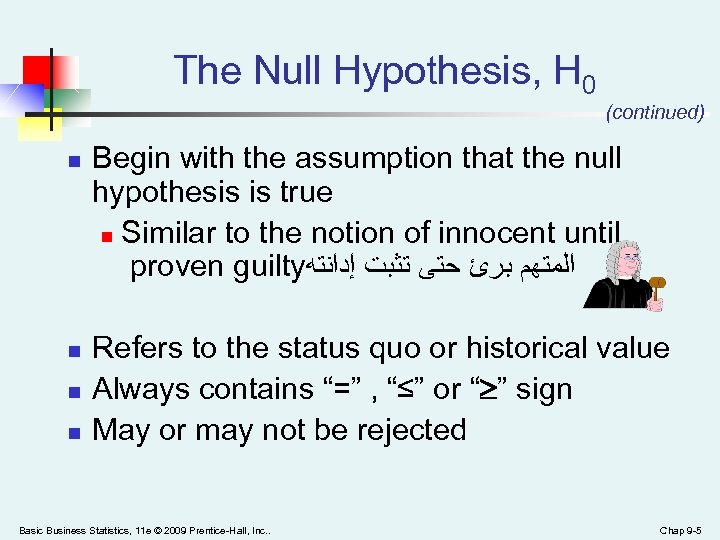The Null Hypothesis, H 0 (continued) n n Begin with the assumption that the null hypothesis is true n Similar to the notion of innocent until proven guilty ﺍﻟﻤﺘﻬﻢ ﺑﺮﺉ ﺣﺘﻰ ﺗﺜﺒﺖ ﺇﺩﺍﻧﺘﻪ Refers to the status quo or historical value Always contains “=” , “≤” or “ ” sign May or may not be rejected Basic Business Statistics, 11 e © 2009 Prentice-Hall, Inc. . Chap 9 -5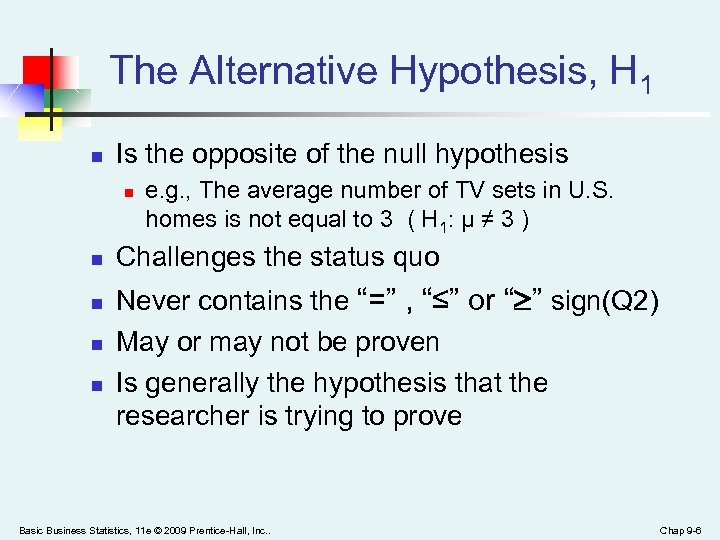The Alternative Hypothesis, H 1 n Is the opposite of the null hypothesis n n n e. g. , The average number of TV sets in U. S. homes is not equal to 3 ( H 1: μ ≠ 3 ) Challenges the status quo Never contains the “=” , “≤” or “ ” sign(Q 2) May or may not be proven Is generally the hypothesis that the researcher is trying to prove Basic Business Statistics, 11 e © 2009 Prentice-Hall, Inc. . Chap 9 -6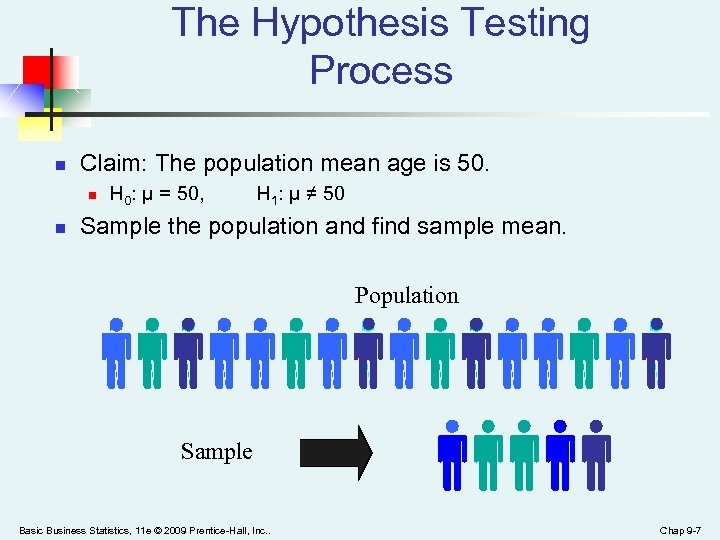The Hypothesis Testing Process n Claim: The population mean age is 50. n n H 0: μ = 50, H 1: μ ≠ 50 Sample the population and find sample mean. Population Sample Basic Business Statistics, 11 e © 2009 Prentice-Hall, Inc. . Chap 9 -7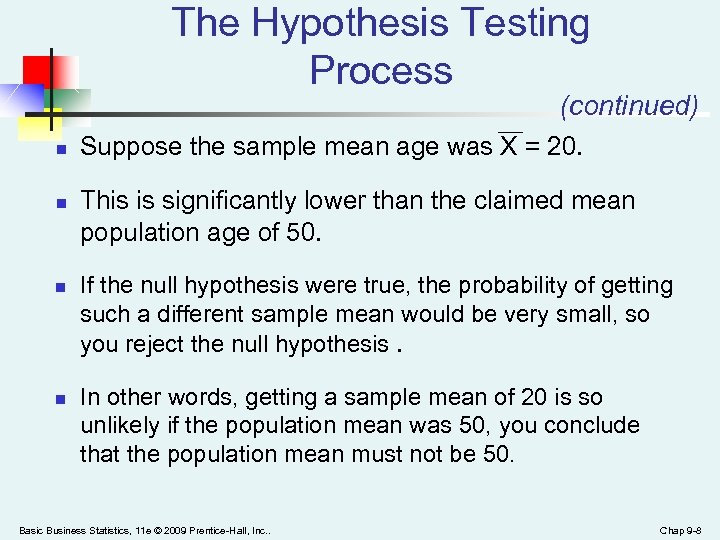The Hypothesis Testing Process (continued) n n Suppose the sample mean age was X = 20. This is significantly lower than the claimed mean population age of 50. If the null hypothesis were true, the probability of getting such a different sample mean would be very small, so you reject the null hypothesis. In other words, getting a sample mean of 20 is so unlikely if the population mean was 50, you conclude that the population mean must not be 50. Basic Business Statistics, 11 e © 2009 Prentice-Hall, Inc. . Chap 9 -8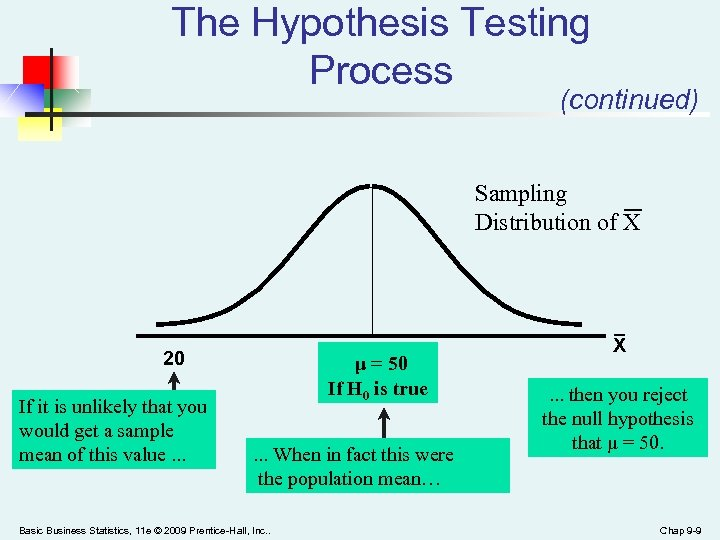The Hypothesis Testing Process (continued) Sampling Distribution of X 20 If it is unlikely that you would get a sample mean of this value. . . μ = 50 If H 0 is true. . . When in fact this were the population mean… Basic Business Statistics, 11 e © 2009 Prentice-Hall, Inc. . X. . . then you reject the null hypothesis that μ = 50. Chap 9 -9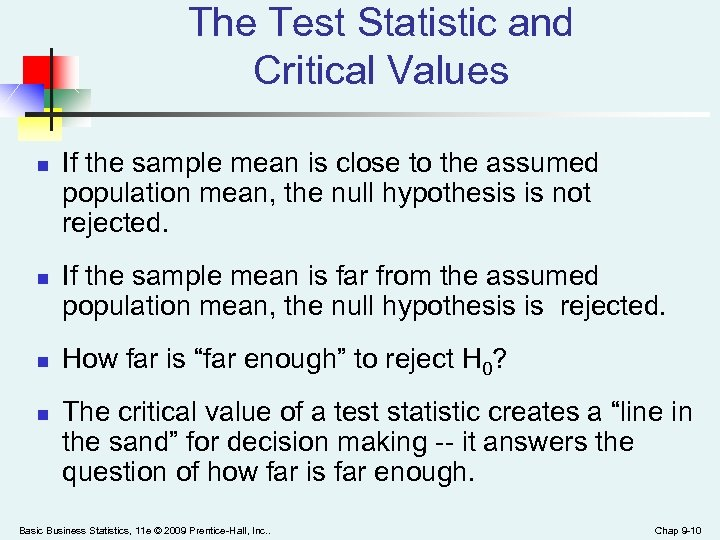The Test Statistic and Critical Values n n If the sample mean is close to the assumed population mean, the null hypothesis is not rejected. If the sample mean is far from the assumed population mean, the null hypothesis is rejected. How far is “far enough” to reject H 0? The critical value of a test statistic creates a “line in the sand” for decision making -- it answers the question of how far is far enough. Basic Business Statistics, 11 e © 2009 Prentice-Hall, Inc. . Chap 9 -10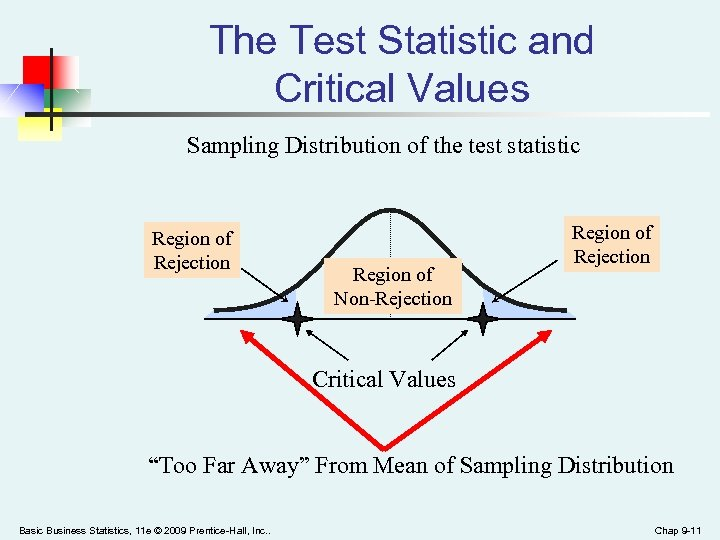The Test Statistic and Critical Values Sampling Distribution of the test statistic Region of Rejection Region of Non-Rejection Region of Rejection Critical Values “Too Far Away” From Mean of Sampling Distribution Basic Business Statistics, 11 e © 2009 Prentice-Hall, Inc. . Chap 9 -11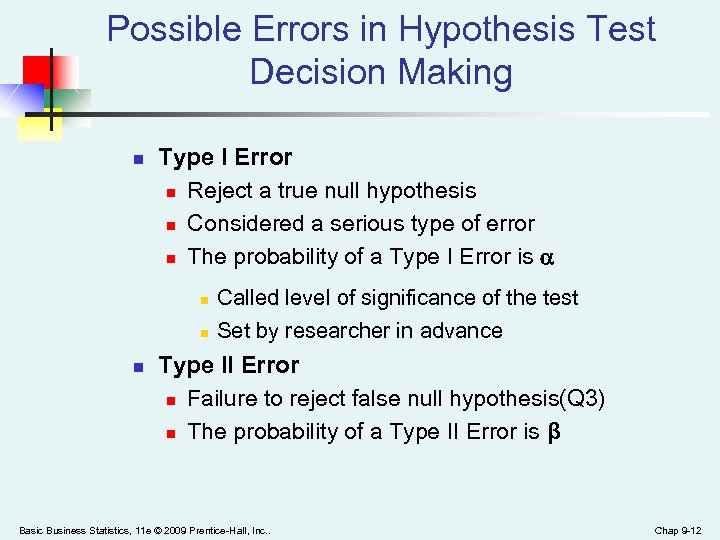Possible Errors in Hypothesis Test Decision Making n Type I Error n Reject a true null hypothesis n Considered a serious type of error n The probability of a Type I Error is n n n Called level of significance of the test Set by researcher in advance Type II Error n Failure to reject false null hypothesis(Q 3) n The probability of a Type II Error is β Basic Business Statistics, 11 e © 2009 Prentice-Hall, Inc. . Chap 9 -12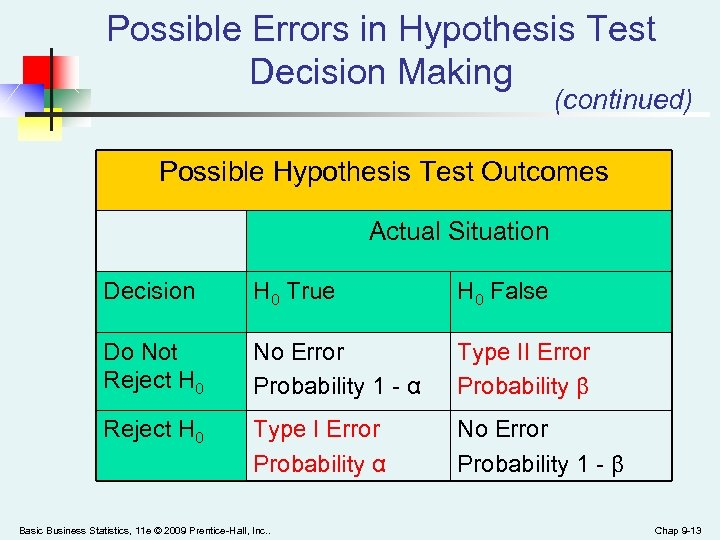Possible Errors in Hypothesis Test Decision Making (continued) Possible Hypothesis Test Outcomes Actual Situation Decision H 0 True H 0 False Do Not Reject H 0 No Error Probability 1 - α Type II Error Probability β Reject H 0 Type I Error Probability α No Error Probability 1 - β Basic Business Statistics, 11 e © 2009 Prentice-Hall, Inc. . Chap 9 -13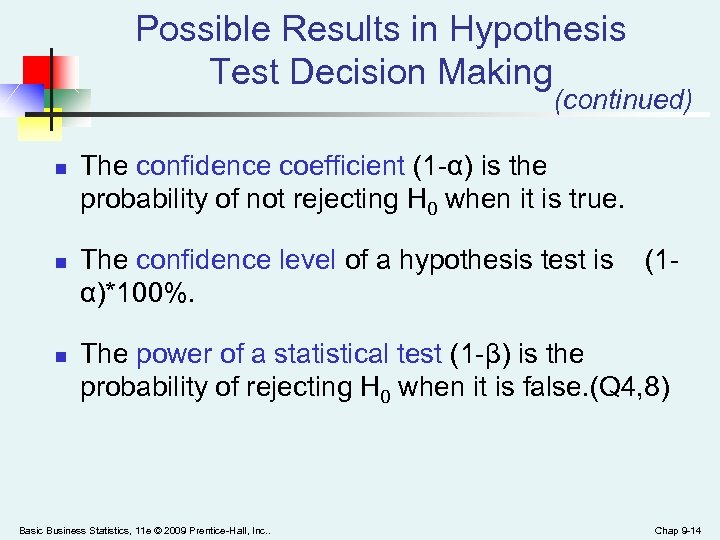Possible Results in Hypothesis Test Decision Making (continued) n n n The confidence coefficient (1 -α) is the probability of not rejecting H 0 when it is true. The confidence level of a hypothesis test is α)*100%. (1 - The power of a statistical test (1 -β) is the probability of rejecting H 0 when it is false. (Q 4, 8) Basic Business Statistics, 11 e © 2009 Prentice-Hall, Inc. . Chap 9 -14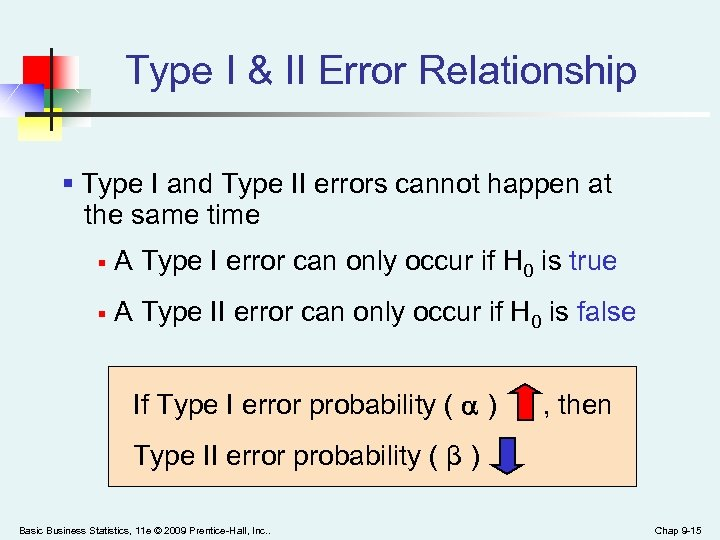Type I & II Error Relationship § Type I and Type II errors cannot happen at the same time § A Type I error can only occur if H 0 is true § A Type II error can only occur if H 0 is false If Type I error probability ( ) , then Type II error probability ( β ) Basic Business Statistics, 11 e © 2009 Prentice-Hall, Inc. . Chap 9 -15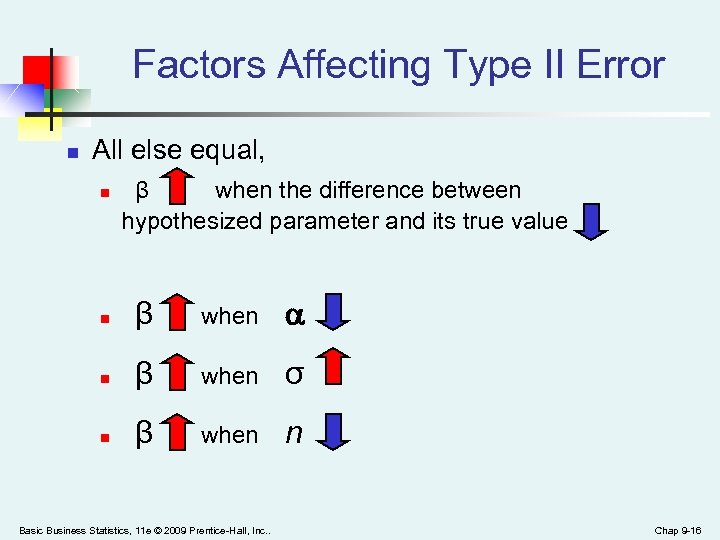Factors Affecting Type II Error n All else equal, n β when the difference between hypothesized parameter and its true value n β when σ n β when n Basic Business Statistics, 11 e © 2009 Prentice-Hall, Inc. . Chap 9 -16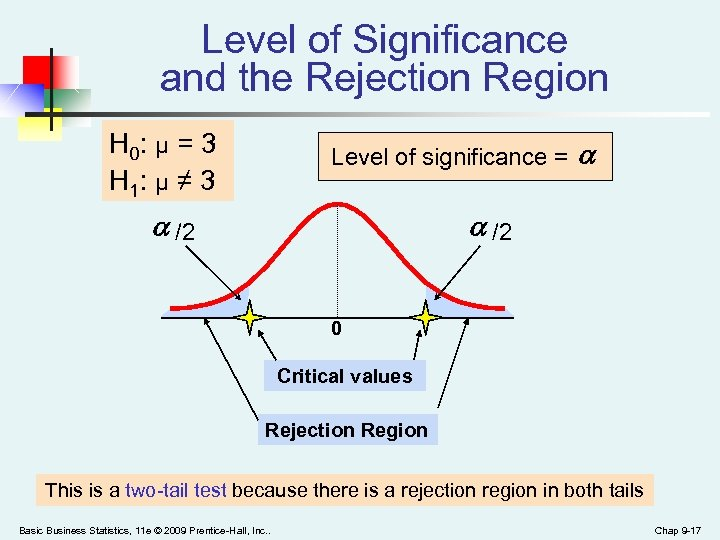Level of Significance and the Rejection Region H 0: μ = 3 H 1: μ ≠ 3 Level of significance = /2 0 Critical values Rejection Region This is a two-tail test because there is a rejection region in both tails Basic Business Statistics, 11 e © 2009 Prentice-Hall, Inc. . Chap 9 -17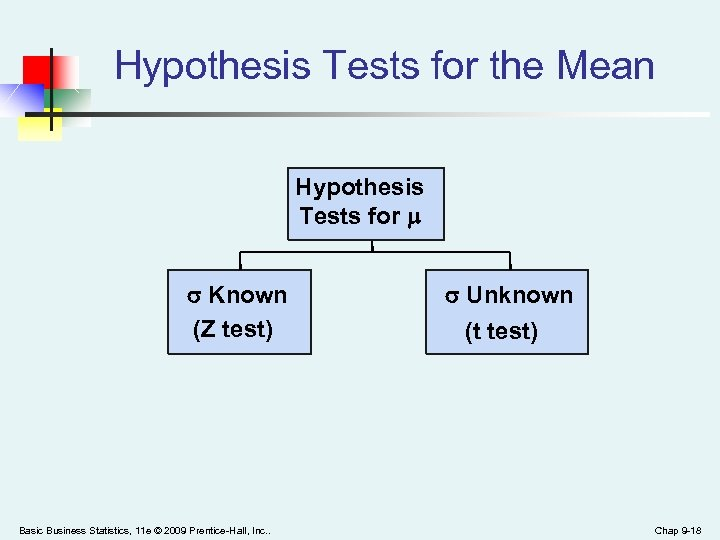Hypothesis Tests for the Mean Hypothesis Tests for Known (Z test) Basic Business Statistics, 11 e © 2009 Prentice-Hall, Inc. . Unknown (t test) Chap 9 -18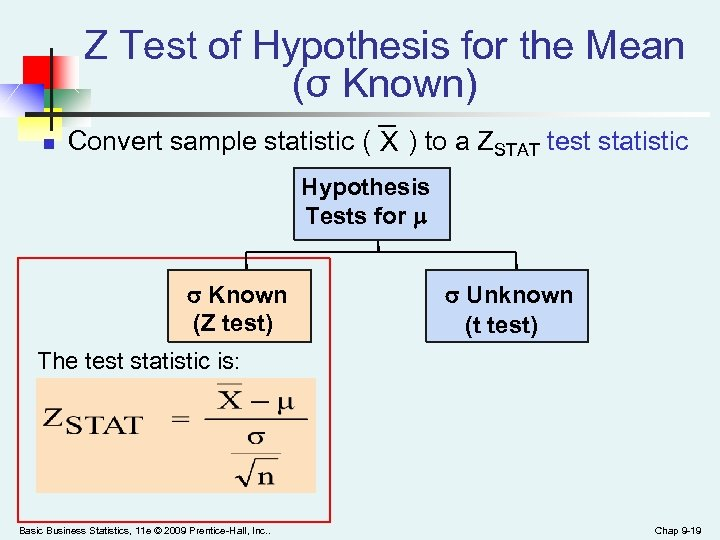Z Test of Hypothesis for the Mean (σ Known) n Convert sample statistic ( X ) to a ZSTAT test statistic Hypothesis Tests for Known σ Known (Z test) Unknown σ Unknown (t test) The test statistic is: Basic Business Statistics, 11 e © 2009 Prentice-Hall, Inc. . Chap 9 -19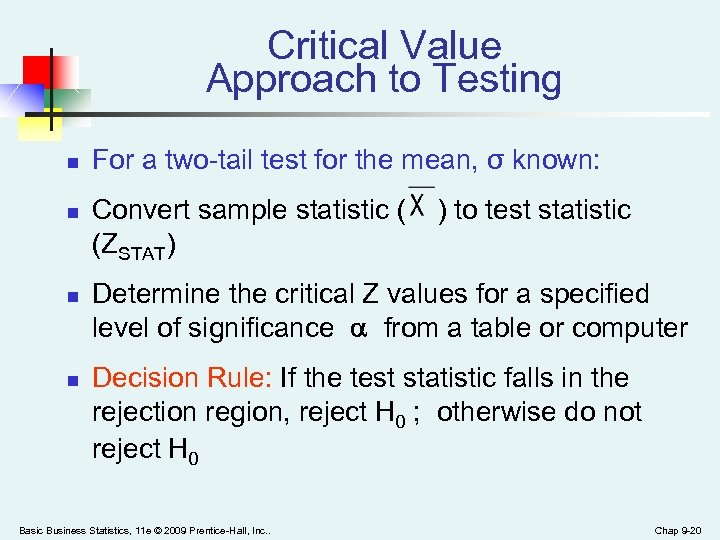Critical Value Approach to Testing n n For a two-tail test for the mean, σ known: Convert sample statistic ( (ZSTAT) ) to test statistic Determine the critical Z values for a specified level of significance from a table or computer Decision Rule: If the test statistic falls in the rejection region, reject H 0 ; otherwise do not reject H 0 Basic Business Statistics, 11 e © 2009 Prentice-Hall, Inc. . Chap 9 -20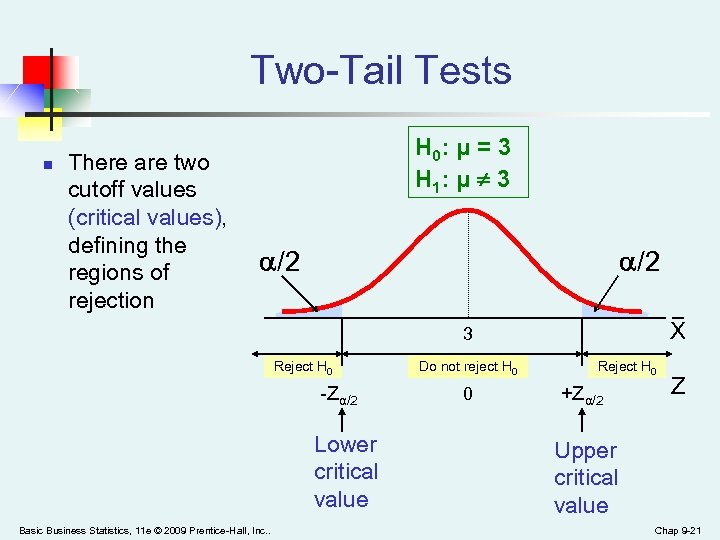Two-Tail Tests n There are two cutoff values (critical values), defining the regions of rejection H 0: μ = 3 H 1: μ ¹ 3 /2 X 3 Reject H 0 -Zα/2 Lower critical value Basic Business Statistics, 11 e © 2009 Prentice-Hall, Inc. . Do not reject H 0 0 Reject H 0 +Zα/2 Z Upper critical value Chap 9 -21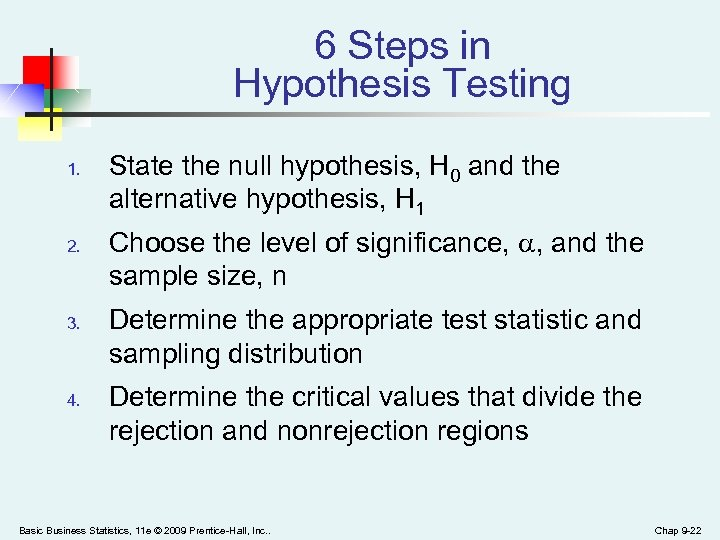6 Steps in Hypothesis Testing 1. 2. 3. 4. State the null hypothesis, H 0 and the alternative hypothesis, H 1 Choose the level of significance, , and the sample size, n Determine the appropriate test statistic and sampling distribution Determine the critical values that divide the rejection and nonrejection regions Basic Business Statistics, 11 e © 2009 Prentice-Hall, Inc. . Chap 9 -22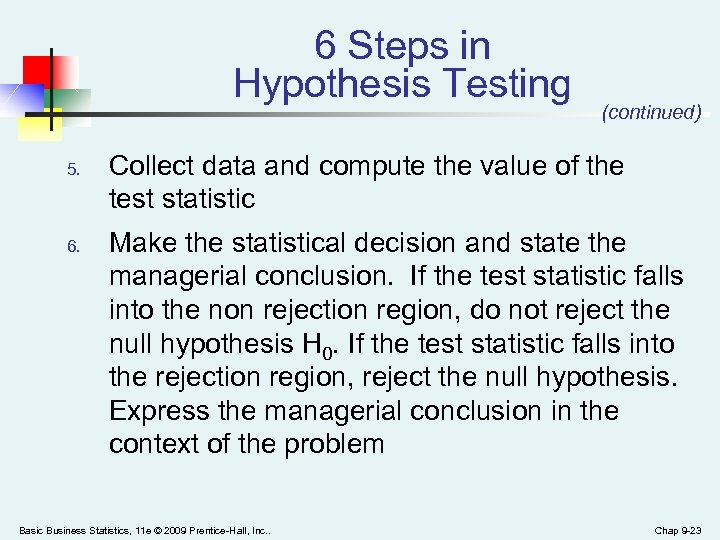6 Steps in Hypothesis Testing 5. 6. (continued) Collect data and compute the value of the test statistic Make the statistical decision and state the managerial conclusion. If the test statistic falls into the non rejection region, do not reject the null hypothesis H 0. If the test statistic falls into the rejection region, reject the null hypothesis. Express the managerial conclusion in the context of the problem Basic Business Statistics, 11 e © 2009 Prentice-Hall, Inc. . Chap 9 -23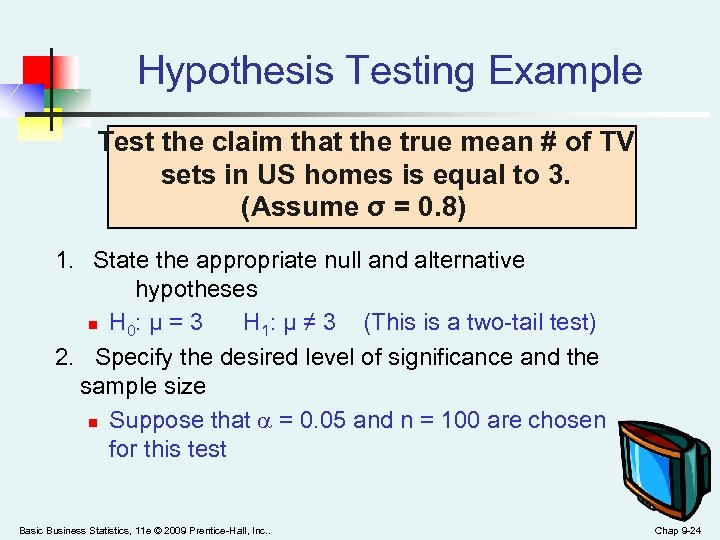Hypothesis Testing Example Test the claim that the true mean # of TV sets in US homes is equal to 3. (Assume σ = 0. 8) 1. State the appropriate null and alternative hypotheses n H 0: μ = 3 H 1: μ ≠ 3 (This is a two-tail test) 2. Specify the desired level of significance and the sample size n Suppose that = 0. 05 and n = 100 are chosen for this test Basic Business Statistics, 11 e © 2009 Prentice-Hall, Inc. . Chap 9 -24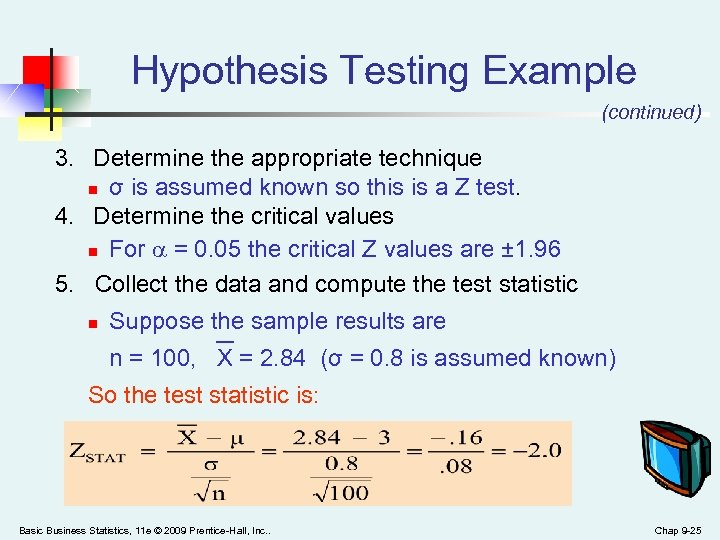Hypothesis Testing Example (continued) 3. Determine the appropriate technique n σ is assumed known so this is a Z test. 4. Determine the critical values n For = 0. 05 the critical Z values are ± 1. 96 5. Collect the data and compute the test statistic n Suppose the sample results are n = 100, X = 2. 84 (σ = 0. 8 is assumed known) So the test statistic is: Basic Business Statistics, 11 e © 2009 Prentice-Hall, Inc. . Chap 9 -25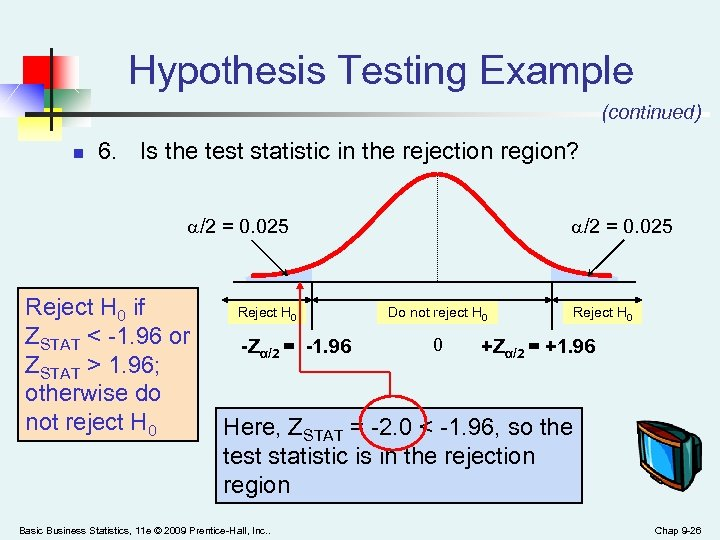Hypothesis Testing Example (continued) n 6. Is the test statistic in the rejection region? /2 = 0. 025 Reject H 0 if ZSTAT < -1. 96 or ZSTAT > 1. 96; otherwise do not reject H 0 Reject H 0 -Zα/2 = -1. 96 /2 = 0. 025 Do not reject H 0 0 Reject H 0 +Zα/2 = +1. 96 Here, ZSTAT = -2. 0 < -1. 96, so the test statistic is in the rejection region Basic Business Statistics, 11 e © 2009 Prentice-Hall, Inc. . Chap 9 -26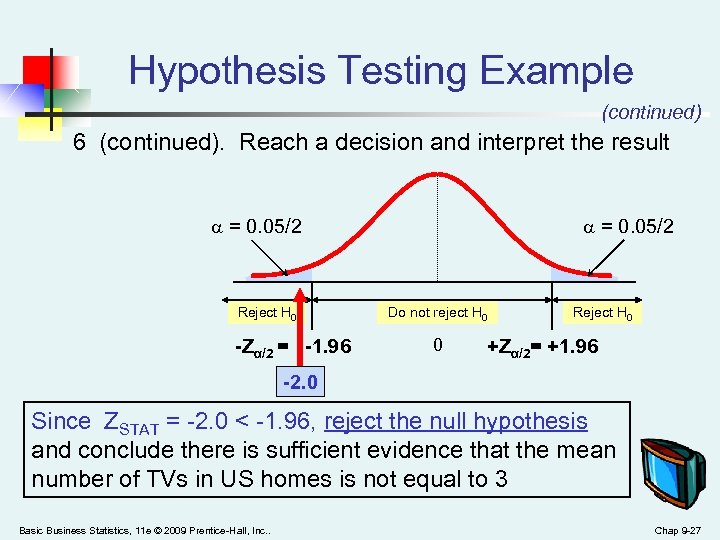Hypothesis Testing Example (continued) 6 (continued). Reach a decision and interpret the result = 0. 05/2 Reject H 0 -Zα/2 = -1. 96 = 0. 05/2 Do not reject H 0 0 Reject H 0 +Zα/2= +1. 96 -2. 0 Since ZSTAT = -2. 0 < -1. 96, reject the null hypothesis and conclude there is sufficient evidence that the mean number of TVs in US homes is not equal to 3 Basic Business Statistics, 11 e © 2009 Prentice-Hall, Inc. . Chap 9 -27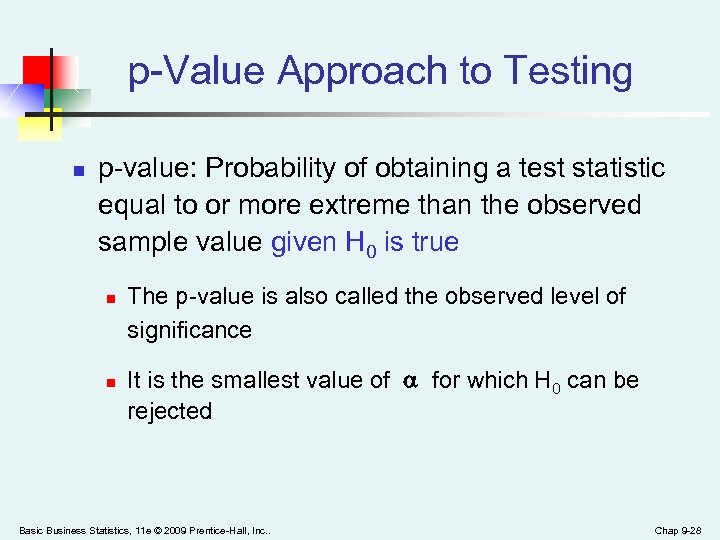p-Value Approach to Testing n p-value: Probability of obtaining a test statistic equal to or more extreme than the observed sample value given H 0 is true n n The p-value is also called the observed level of significance It is the smallest value of for which H 0 can be rejected Basic Business Statistics, 11 e © 2009 Prentice-Hall, Inc. . Chap 9 -28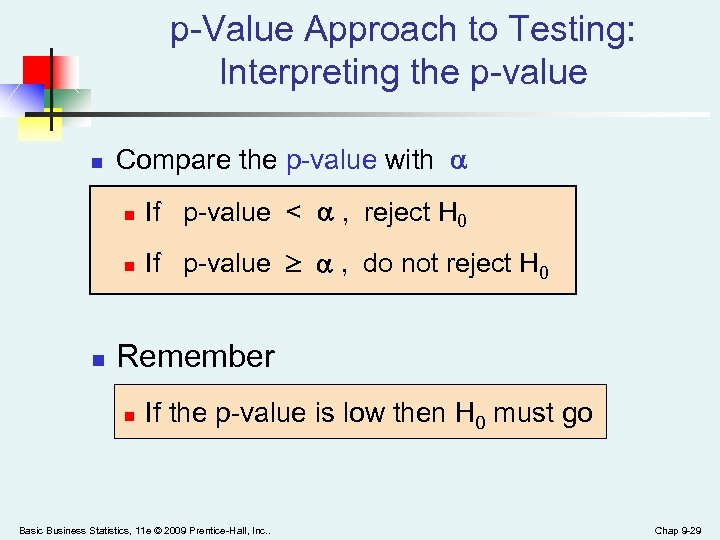p-Value Approach to Testing: Interpreting the p-value n Compare the p-value with n n n If p-value < , reject H 0 If p-value , do not reject H 0 Remember n If the p-value is low then H 0 must go Basic Business Statistics, 11 e © 2009 Prentice-Hall, Inc. . Chap 9 -29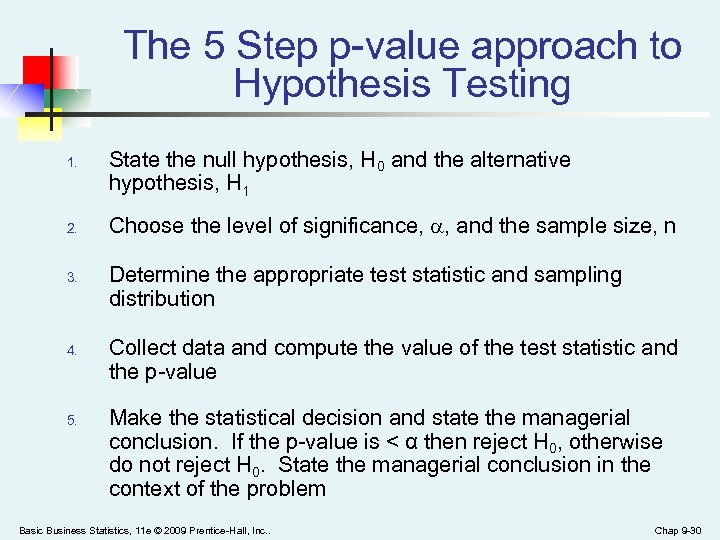The 5 Step p-value approach to Hypothesis Testing 1. 2. 3. 4. 5. State the null hypothesis, H 0 and the alternative hypothesis, H 1 Choose the level of significance, , and the sample size, n Determine the appropriate test statistic and sampling distribution Collect data and compute the value of the test statistic and the p-value Make the statistical decision and state the managerial conclusion. If the p-value is < α then reject H 0, otherwise do not reject H 0. State the managerial conclusion in the context of the problem Basic Business Statistics, 11 e © 2009 Prentice-Hall, Inc. . Chap 9 -30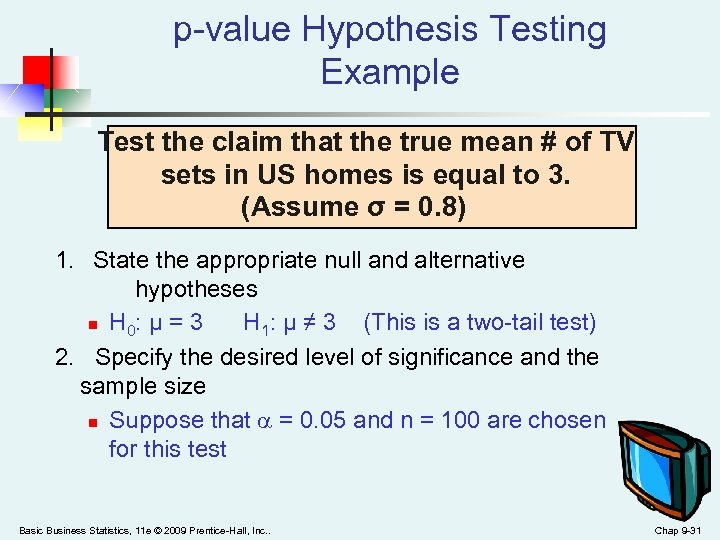p-value Hypothesis Testing Example Test the claim that the true mean # of TV sets in US homes is equal to 3. (Assume σ = 0. 8) 1. State the appropriate null and alternative hypotheses n H 0: μ = 3 H 1: μ ≠ 3 (This is a two-tail test) 2. Specify the desired level of significance and the sample size n Suppose that = 0. 05 and n = 100 are chosen for this test Basic Business Statistics, 11 e © 2009 Prentice-Hall, Inc. . Chap 9 -31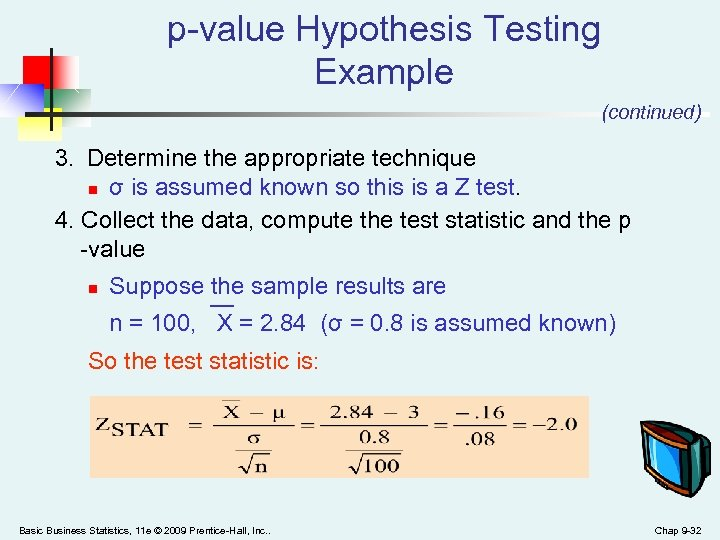p-value Hypothesis Testing Example (continued) 3. Determine the appropriate technique n σ is assumed known so this is a Z test. 4. Collect the data, compute the test statistic and the p -value n Suppose the sample results are n = 100, X = 2. 84 (σ = 0. 8 is assumed known) So the test statistic is: Basic Business Statistics, 11 e © 2009 Prentice-Hall, Inc. . Chap 9 -32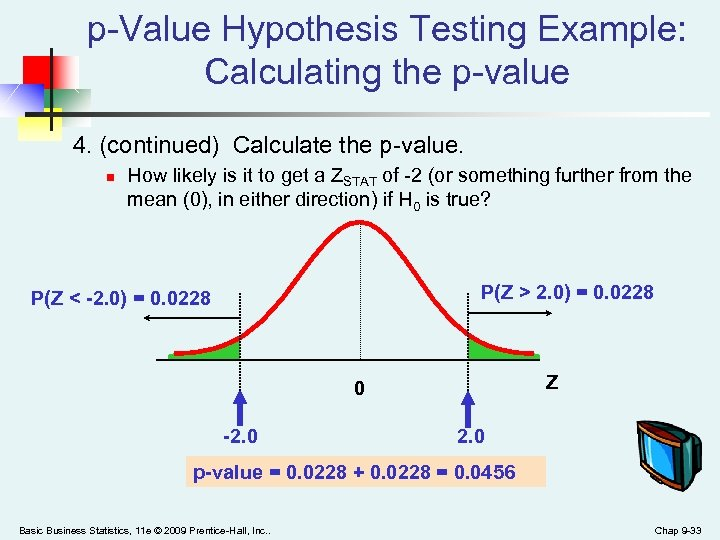p-Value Hypothesis Testing Example: Calculating the p-value 4. (continued) Calculate the p-value. n How likely is it to get a ZSTAT of -2 (or something further from the mean (0), in either direction) if H 0 is true? P(Z > 2. 0) = 0. 0228 P(Z < -2. 0) = 0. 0228 Z 0 -2. 0 p-value = 0. 0228 + 0. 0228 = 0. 0456 Basic Business Statistics, 11 e © 2009 Prentice-Hall, Inc. . Chap 9 -33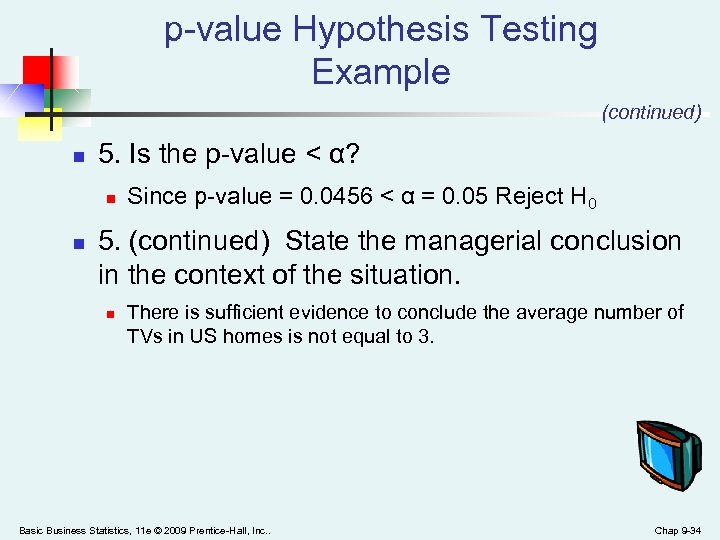p-value Hypothesis Testing Example (continued) n 5. Is the p-value < α? n n Since p-value = 0. 0456 < α = 0. 05 Reject H 0 5. (continued) State the managerial conclusion in the context of the situation. n There is sufficient evidence to conclude the average number of TVs in US homes is not equal to 3. Basic Business Statistics, 11 e © 2009 Prentice-Hall, Inc. . Chap 9 -34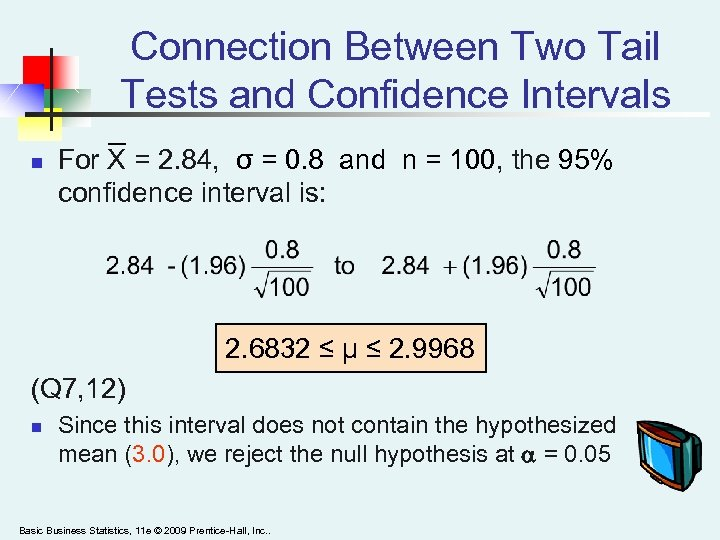Connection Between Two Tail Tests and Confidence Intervals n For X = 2. 84, σ = 0. 8 and n = 100, the 95% confidence interval is: 2. 6832 ≤ μ ≤ 2. 9968 (Q 7, 12) n Since this interval does not contain the hypothesized mean (3. 0), we reject the null hypothesis at = 0. 05 Basic Business Statistics, 11 e © 2009 Prentice-Hall, Inc. .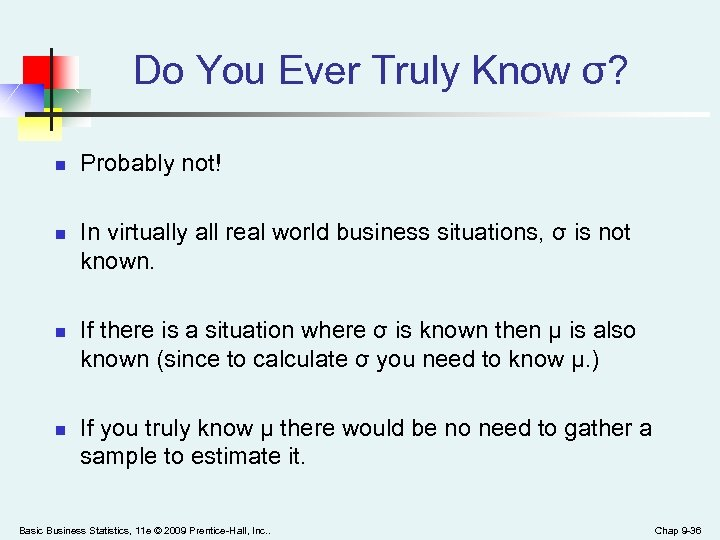Do You Ever Truly Know σ? n n Probably not! In virtually all real world business situations, σ is not known. If there is a situation where σ is known then µ is also known (since to calculate σ you need to know µ. ) If you truly know µ there would be no need to gather a sample to estimate it. Basic Business Statistics, 11 e © 2009 Prentice-Hall, Inc. . Chap 9 -36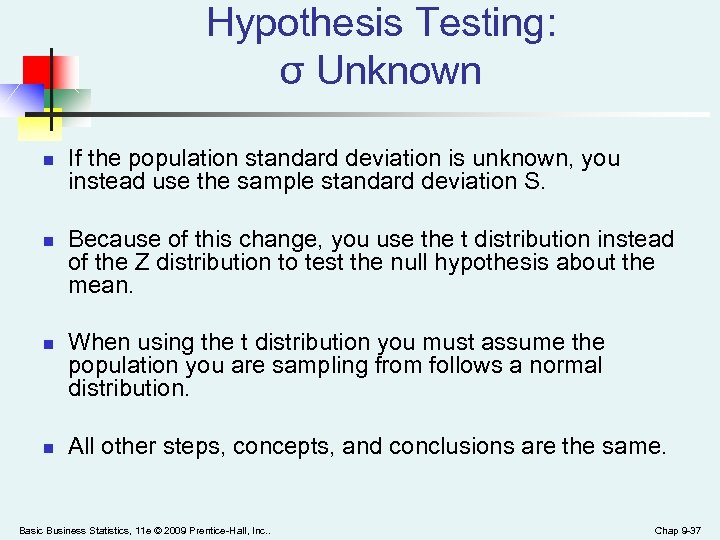Hypothesis Testing: σ Unknown n n If the population standard deviation is unknown, you instead use the sample standard deviation S. Because of this change, you use the t distribution instead of the Z distribution to test the null hypothesis about the mean. When using the t distribution you must assume the population you are sampling from follows a normal distribution. All other steps, concepts, and conclusions are the same. Basic Business Statistics, 11 e © 2009 Prentice-Hall, Inc. . Chap 9 -37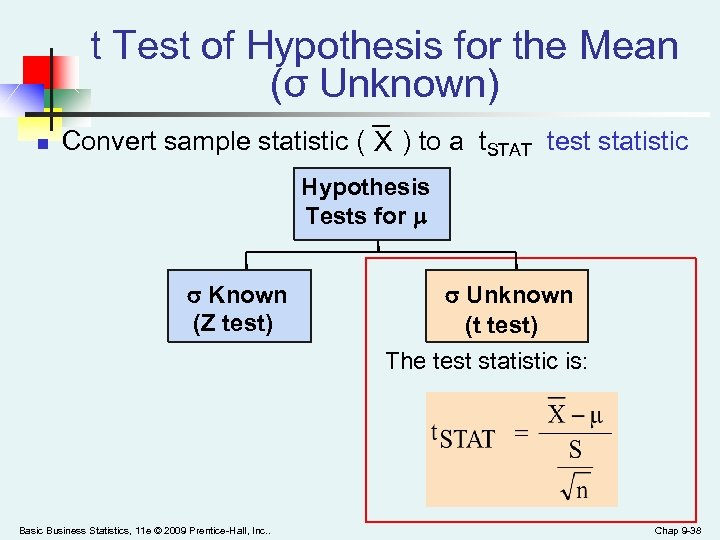t Test of Hypothesis for the Mean (σ Unknown) n Convert sample statistic ( X ) to a t. STAT test statistic Hypothesis Tests for Known σ Known (Z test) Basic Business Statistics, 11 e © 2009 Prentice-Hall, Inc. . Unknown σ Unknown (t test) The test statistic is: Chap 9 -38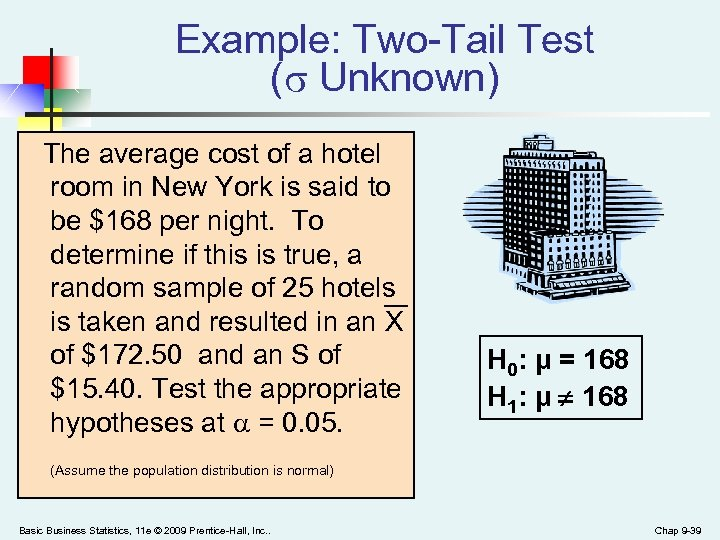Example: Two-Tail Test ( Unknown) The average cost of a hotel room in New York is said to be \$168 per night. To determine if this is true, a random sample of 25 hotels is taken and resulted in an X of \$172. 50 and an S of \$15. 40. Test the appropriate hypotheses at = 0. 05. H 0: μ = 168 H 1: μ ¹ 168 (Assume the population distribution is normal) Basic Business Statistics, 11 e © 2009 Prentice-Hall, Inc. . Chap 9 -39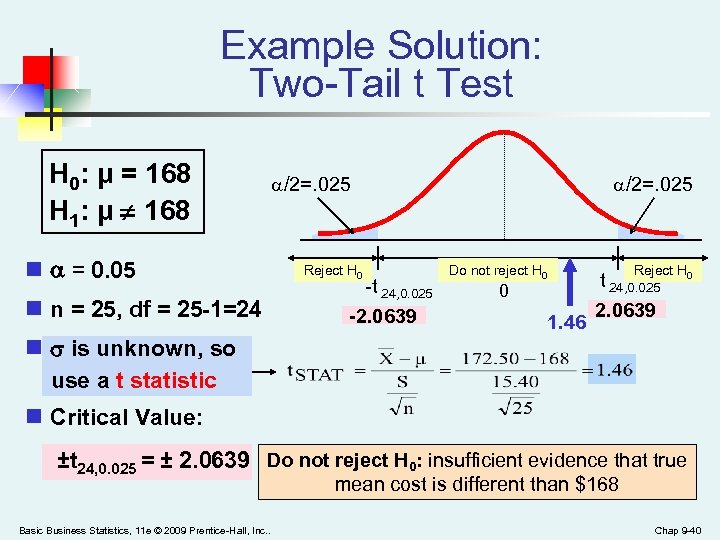Example Solution: Two-Tail t Test H 0: μ = 168 H 1: μ ¹ 168 n = 0. 05 n n = 25, df = 25 -1=24 n is unknown, so use a t statistic /2=. 025 Reject H 0 -t 24, 0. 025 -2. 0639 /2=. 025 Do not reject H 0 0 1. 46 Reject H 0 t 24, 0. 025 2. 0639 n Critical Value: ±t 24, 0. 025 = ± 2. 0639 Do not reject H 0: insufficient evidence that true mean cost is different than \$168 Basic Business Statistics, 11 e © 2009 Prentice-Hall, Inc. . Chap 9 -40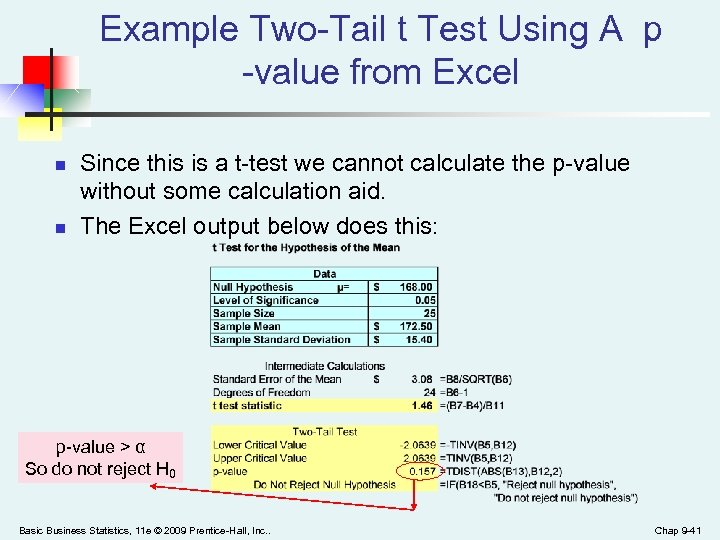Example Two-Tail t Test Using A p -value from Excel n n Since this is a t-test we cannot calculate the p-value without some calculation aid. The Excel output below does this: p-value > α So do not reject H 0 Basic Business Statistics, 11 e © 2009 Prentice-Hall, Inc. . Chap 9 -41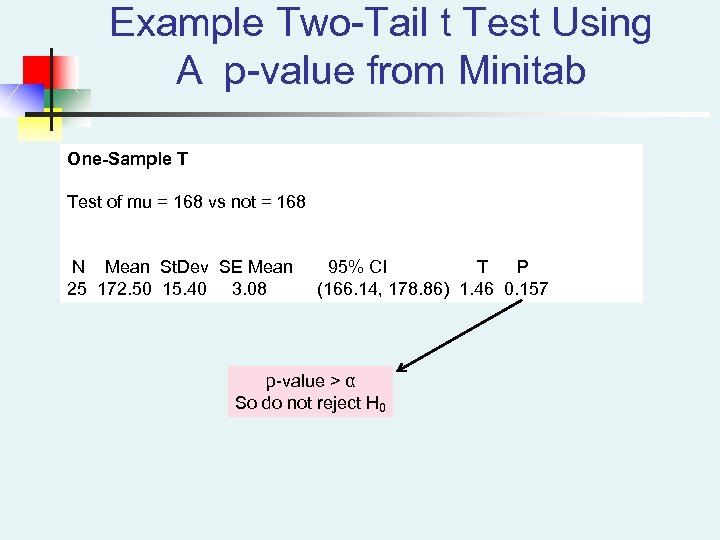Example Two-Tail t Test Using A p-value from Minitab One-Sample T Test of mu = 168 vs not = 168 N Mean St. Dev SE Mean 25 172. 50 15. 40 3. 08 95% CI T P (166. 14, 178. 86) 1. 46 0. 157 p-value > α So do not reject H 0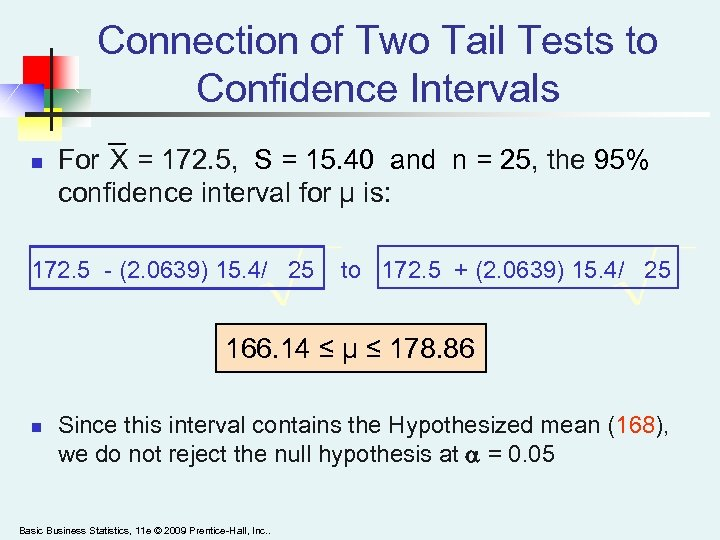Connection of Two Tail Tests to Confidence Intervals n For X = 172. 5, S = 15. 40 and n = 25, the 95% confidence interval for µ is: 172. 5 - (2. 0639) 15. 4/ 25 to 172. 5 + (2. 0639) 15. 4/ 25 166. 14 ≤ μ ≤ 178. 86 n Since this interval contains the Hypothesized mean (168), we do not reject the null hypothesis at = 0. 05 Basic Business Statistics, 11 e © 2009 Prentice-Hall, Inc. .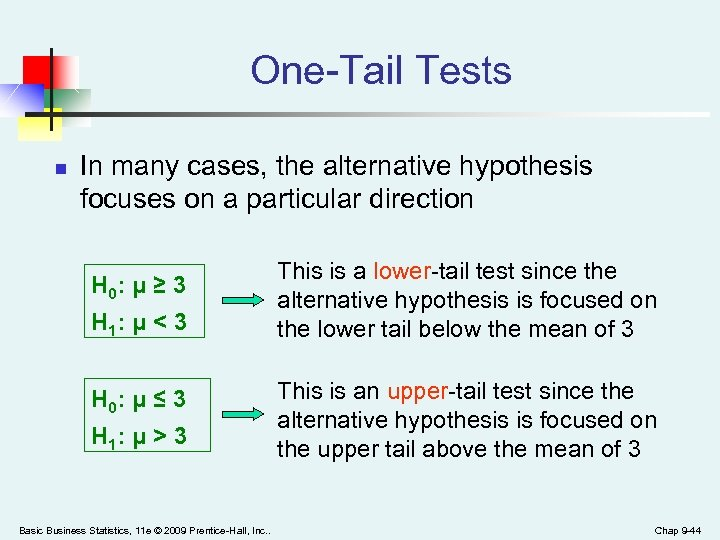One-Tail Tests n In many cases, the alternative hypothesis focuses on a particular direction H 0: μ ≥ 3 H 1: μ < 3 H 0: μ ≤ 3 H 1: μ > 3 Basic Business Statistics, 11 e © 2009 Prentice-Hall, Inc. . This is a lower-tail test since the alternative hypothesis is focused on the lower tail below the mean of 3 This is an upper-tail test since the alternative hypothesis is focused on the upper tail above the mean of 3 Chap 9 -44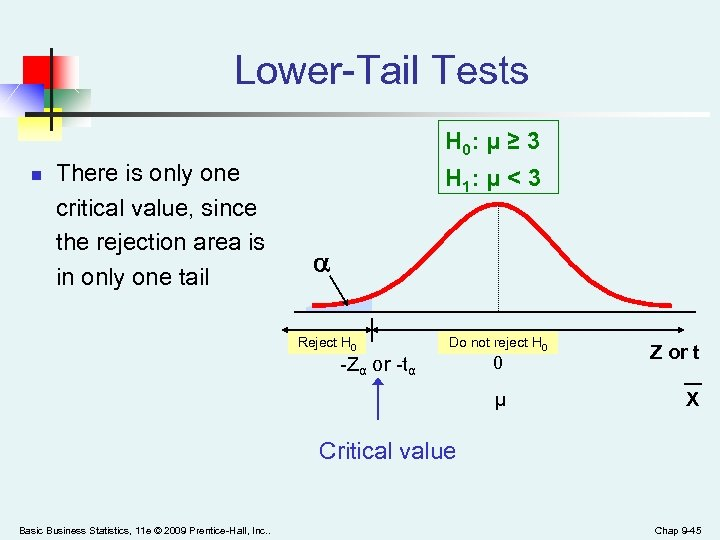Lower-Tail Tests H 0: μ ≥ 3 n There is only one critical value, since the rejection area is in only one tail H 1: μ < 3 Reject H 0 Do not reject H 0 -Zα or -tα 0 μ Z or t X Critical value Basic Business Statistics, 11 e © 2009 Prentice-Hall, Inc. . Chap 9 -45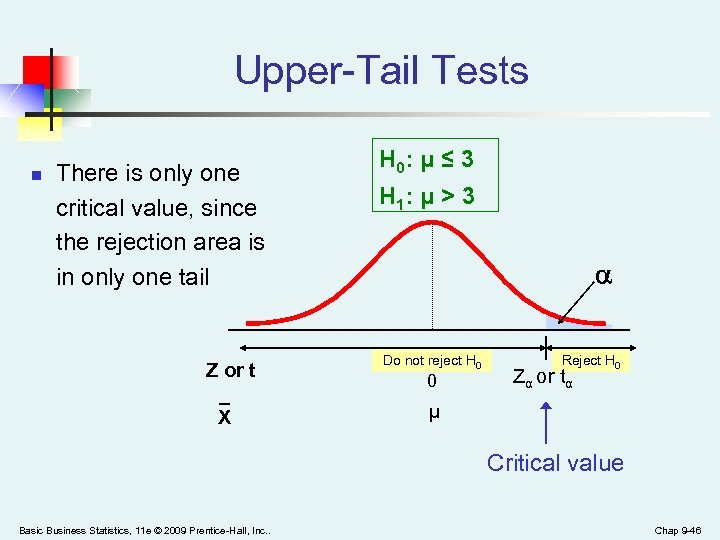Upper-Tail Tests n There is only one critical value, since the rejection area is in only one tail Z or t _ X H 0: μ ≤ 3 H 1: μ > 3 Do not reject H 0 0 Reject H 0 Zα or tα μ Critical value Basic Business Statistics, 11 e © 2009 Prentice-Hall, Inc. . Chap 9 -46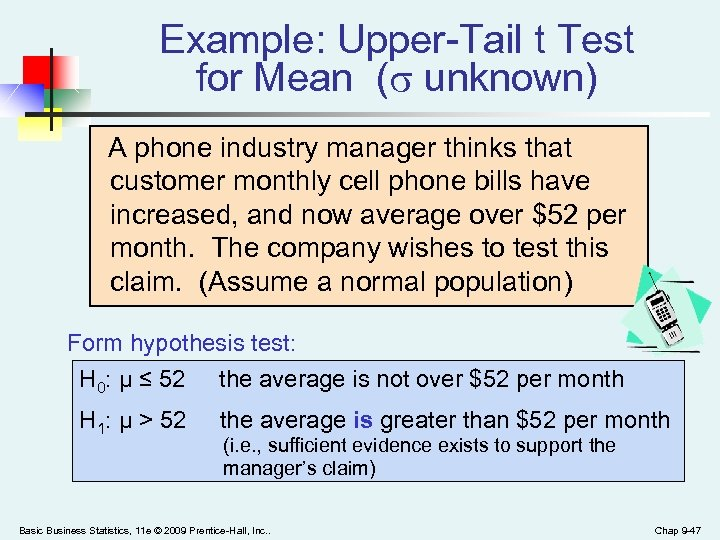Example: Upper-Tail t Test for Mean ( unknown) A phone industry manager thinks that customer monthly cell phone bills have increased, and now average over \$52 per month. The company wishes to test this claim. (Assume a normal population) Form hypothesis test: H 0: μ ≤ 52 the average is not over \$52 per month H 1: μ > 52 the average is greater than \$52 per month (i. e. , sufficient evidence exists to support the manager’s claim) Basic Business Statistics, 11 e © 2009 Prentice-Hall, Inc. . Chap 9 -47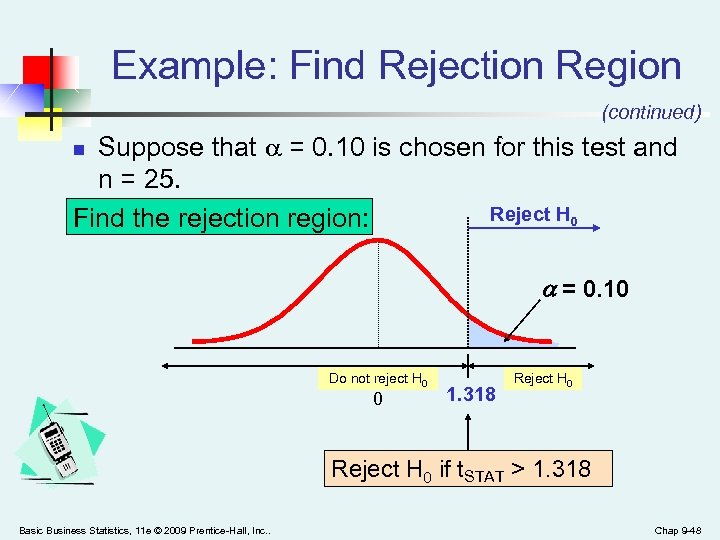Example: Find Rejection Region (continued) Suppose that = 0. 10 is chosen for this test and n = 25. Reject H 0 Find the rejection region: n = 0. 10 Do not reject H 0 0 1. 318 Reject H 0 if t. STAT > 1. 318 Basic Business Statistics, 11 e © 2009 Prentice-Hall, Inc. . Chap 9 -48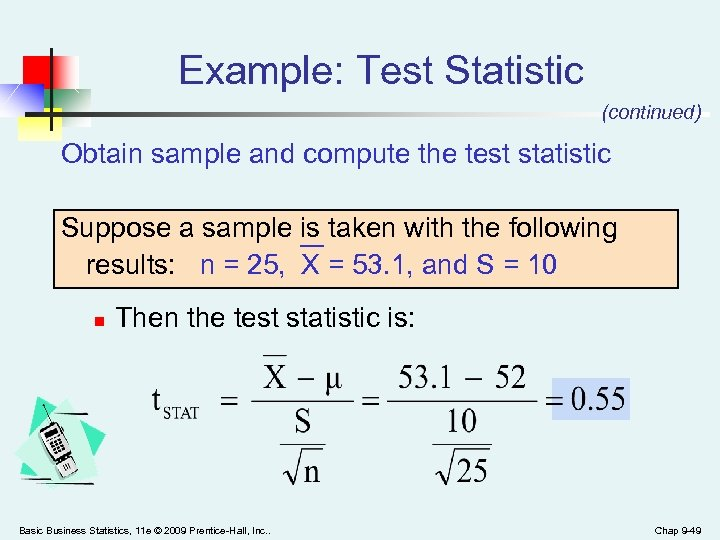Example: Test Statistic (continued) Obtain sample and compute the test statistic Suppose a sample is taken with the following results: n = 25, X = 53. 1, and S = 10 n Then the test statistic is: Basic Business Statistics, 11 e © 2009 Prentice-Hall, Inc. . Chap 9 -49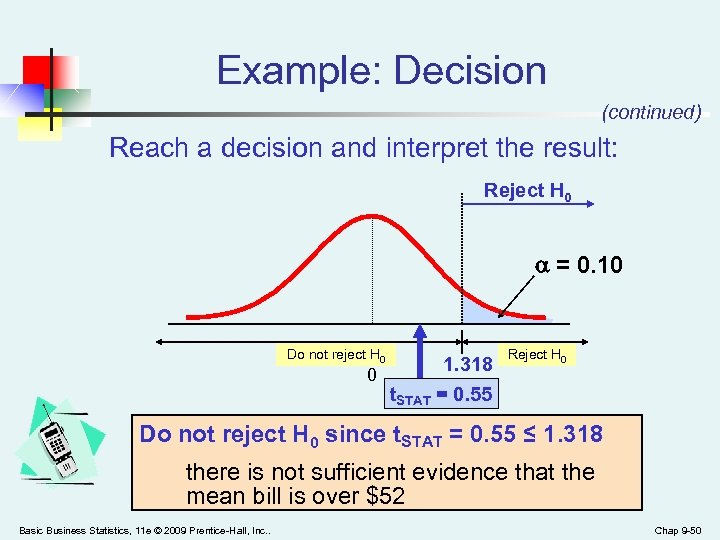Example: Decision (continued) Reach a decision and interpret the result: Reject H 0 = 0. 10 Do not reject H 0 1. 318 0 t. STAT = 0. 55 Reject H 0 Do not reject H 0 since t. STAT = 0. 55 ≤ 1. 318 there is not sufficient evidence that the mean bill is over \$52 Basic Business Statistics, 11 e © 2009 Prentice-Hall, Inc. . Chap 9 -50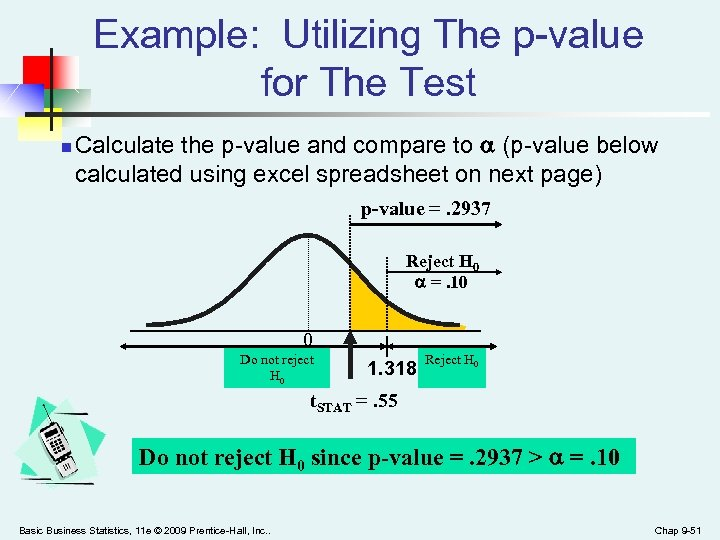Example: Utilizing The p-value for The Test n Calculate the p-value and compare to (p-value below calculated using excel spreadsheet on next page) p-value =. 2937 Reject H 0 =. 10 0 Do not reject H 0 1. 318 Reject H 0 t. STAT =. 55 Do not reject H 0 since p-value =. 2937 > =. 10 Basic Business Statistics, 11 e © 2009 Prentice-Hall, Inc. . Chap 9 -51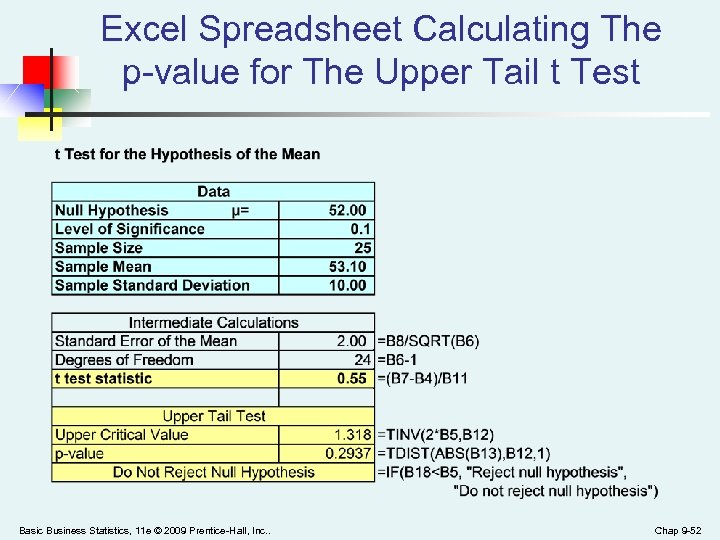Excel Spreadsheet Calculating The p-value for The Upper Tail t Test Basic Business Statistics, 11 e © 2009 Prentice-Hall, Inc. . Chap 9 -52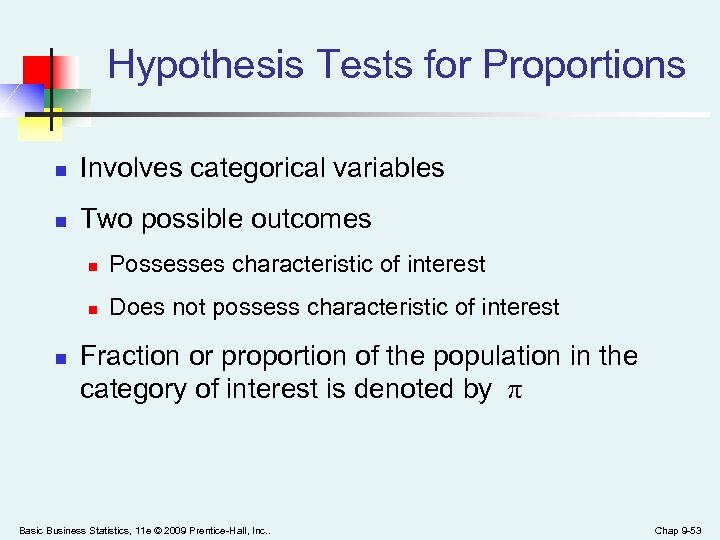Hypothesis Tests for Proportions n Involves categorical variables n Two possible outcomes n n n Possesses characteristic of interest Does not possess characteristic of interest Fraction or proportion of the population in the category of interest is denoted by π Basic Business Statistics, 11 e © 2009 Prentice-Hall, Inc. . Chap 9 -53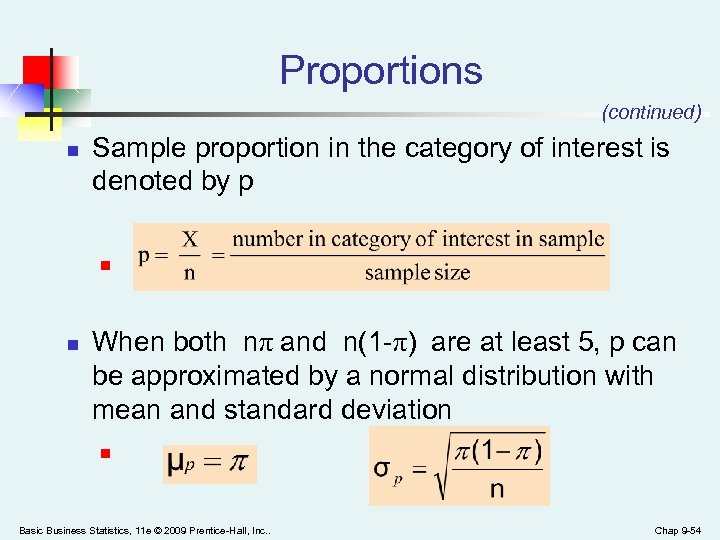Proportions (continued) n Sample proportion in the category of interest is denoted by p n n When both nπ and n(1 -π) are at least 5, p can be approximated by a normal distribution with mean and standard deviation n Basic Business Statistics, 11 e © 2009 Prentice-Hall, Inc. . Chap 9 -54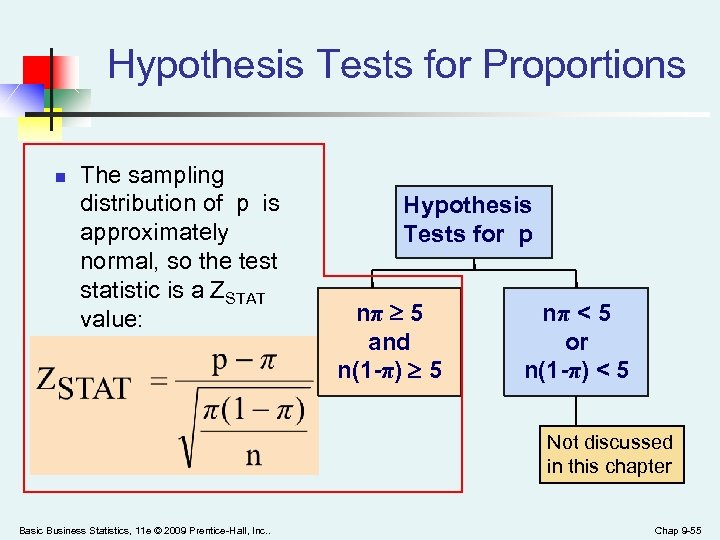Hypothesis Tests for Proportions n The sampling distribution of p is approximately normal, so the test statistic is a ZSTAT value: Hypothesis Tests for p nπ 5 and n(1 -π) 5 nπ < 5 or n(1 -π) < 5 Not discussed in this chapter Basic Business Statistics, 11 e © 2009 Prentice-Hall, Inc. . Chap 9 -55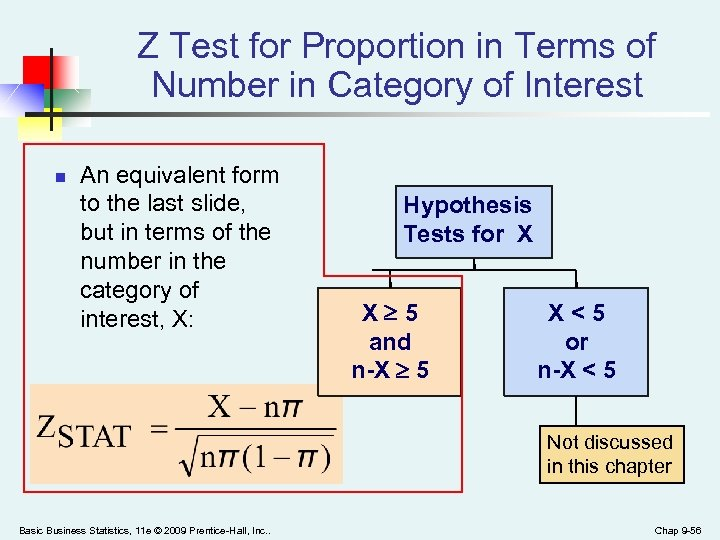Z Test for Proportion in Terms of Number in Category of Interest n An equivalent form to the last slide, but in terms of the number in the category of interest, X: Hypothesis Tests for X X 5 and n-X 5 X<5 or n-X < 5 Not discussed in this chapter Basic Business Statistics, 11 e © 2009 Prentice-Hall, Inc. . Chap 9 -56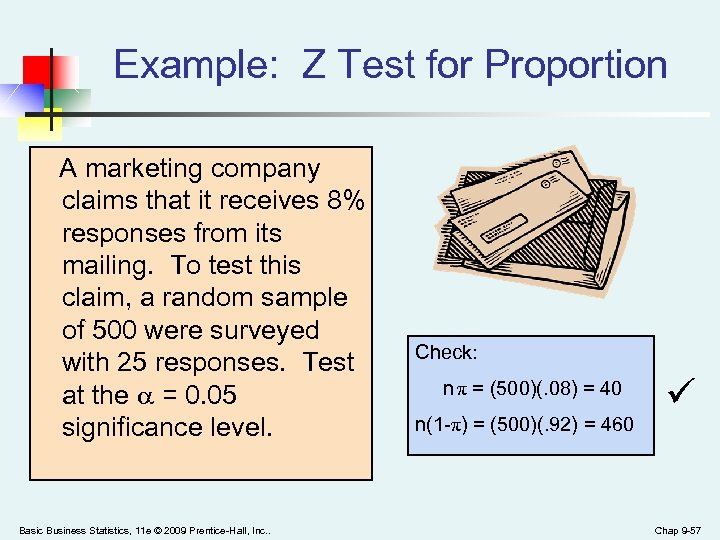Example: Z Test for Proportion A marketing company claims that it receives 8% responses from its mailing. To test this claim, a random sample of 500 were surveyed with 25 responses. Test at the = 0. 05 significance level. Basic Business Statistics, 11 e © 2009 Prentice-Hall, Inc. . Check: n π = (500)(. 08) = 40 n(1 -π) = (500)(. 92) = 460 Chap 9 -57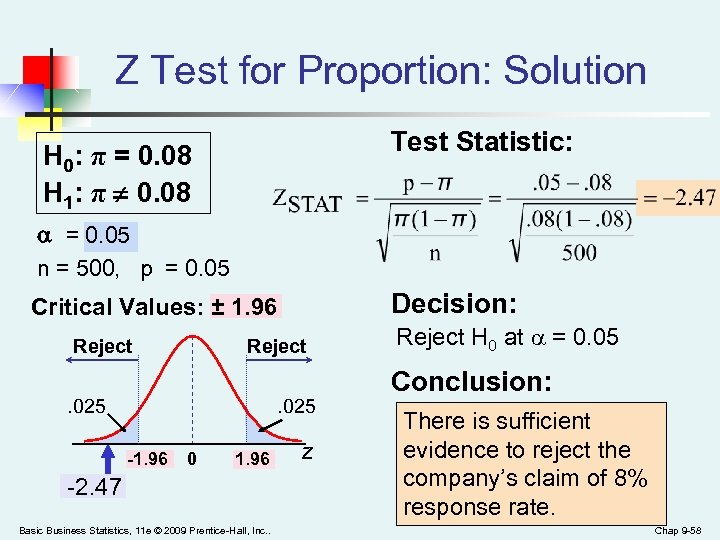Z Test for Proportion: Solution Test Statistic: H 0: π = 0. 08 H 1: π ¹ 0. 08 = 0. 05 n = 500, p = 0. 05 Decision: Critical Values: ± 1. 96 Reject . 025 -1. 96 0 1. 96 -2. 47 Basic Business Statistics, 11 e © 2009 Prentice-Hall, Inc. . z Reject H 0 at = 0. 05 Conclusion: There is sufficient evidence to reject the company’s claim of 8% response rate. Chap 9 -58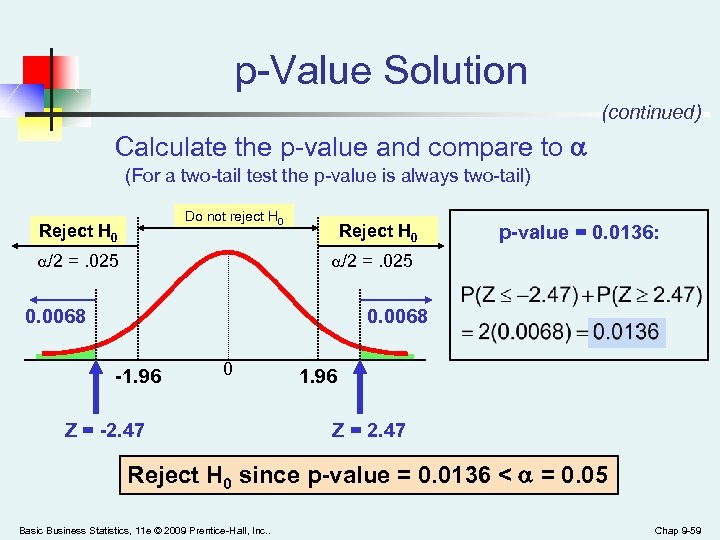p-Value Solution (continued) Calculate the p-value and compare to (For a two-tail test the p-value is always two-tail) Do not reject H 0 Reject H 0 /2 =. 025 Reject H 0 p-value = 0. 0136: /2 =. 025 0. 0068 -1. 96 0 Z = -2. 47 1. 96 Z = 2. 47 Reject H 0 since p-value = 0. 0136 < = 0. 05 Basic Business Statistics, 11 e © 2009 Prentice-Hall, Inc. . Chap 9 -59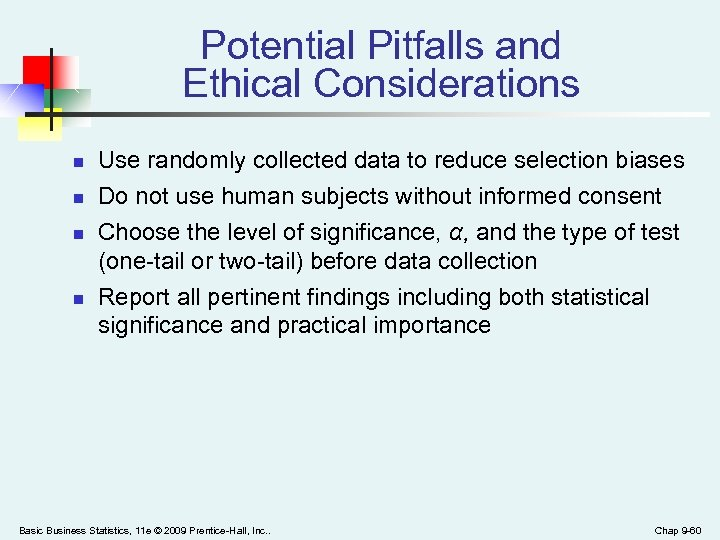Potential Pitfalls and Ethical Considerations n n Use randomly collected data to reduce selection biases Do not use human subjects without informed consent Choose the level of significance, α, and the type of test (one-tail or two-tail) before data collection Report all pertinent findings including both statistical significance and practical importance Basic Business Statistics, 11 e © 2009 Prentice-Hall, Inc. . Chap 9 -60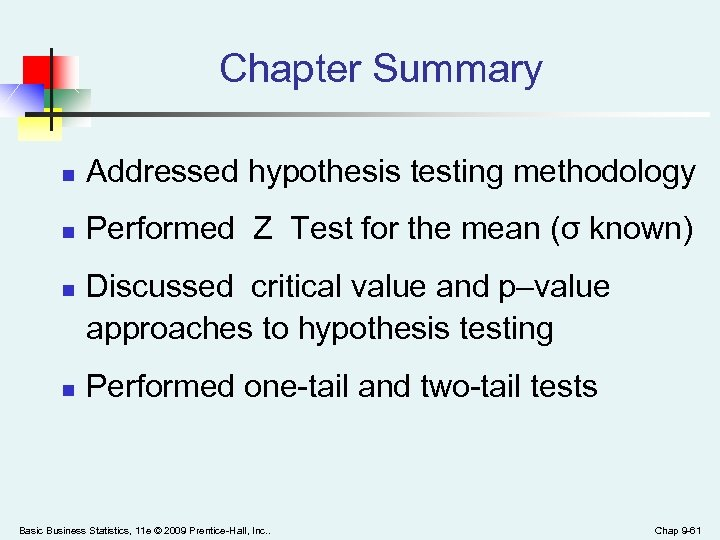Chapter Summary n Addressed hypothesis testing methodology n Performed Z Test for the mean (σ known) n n Discussed critical value and p–value approaches to hypothesis testing Performed one-tail and two-tail tests Basic Business Statistics, 11 e © 2009 Prentice-Hall, Inc. . Chap 9 -61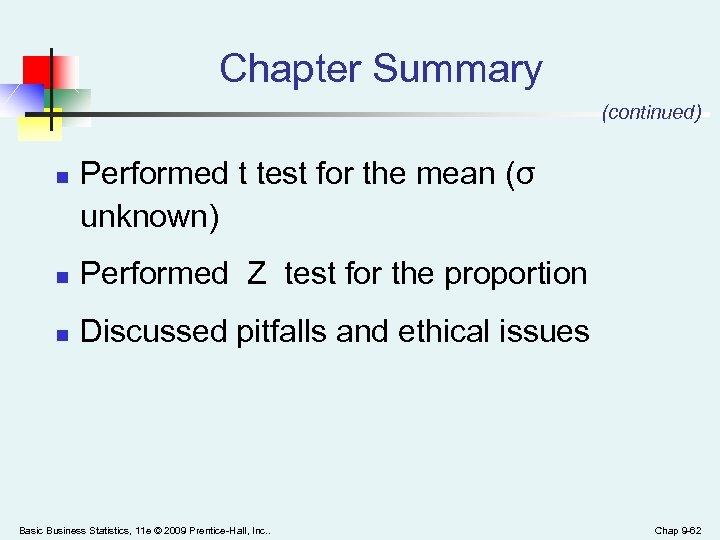Chapter Summary (continued) n Performed t test for the mean (σ unknown) n Performed Z test for the proportion n Discussed pitfalls and ethical issues Basic Business Statistics, 11 e © 2009 Prentice-Hall, Inc. . Chap 9 -62